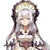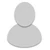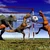25,991 Pages

## What is Inverse Square Law?

Wikipedia describes Inverse Square Law as this:

 “ In physics, an inverse-square law is any physical law stating that a specified physical quantity or intensity is inversely proportional to the square of the distance from the source of that physical quantity. „

A simplified formula for this is area of the larger object or explosion/area of the object x the initial value

It can be used for many different subjects:

### Example 1: Finding energy based on something destroyed within an explosion

A ground explosion with a radius of 5 meters has exactly enough energy so when it hits a brick with an area of 0.07116953508 square meters and a volume of 0.0010692559 cubic meters, it vaporizes it. How much energy does this explosion hold?

• First we use the known value for vaporization onto the brick, which is 25700 joules per cubic centimeter. It requires 27.4798773 megajoules to vaporize said brick.
• When dealing with ground explosions, one should use a hemisphere as a basis for the explosion’s shape. The area of a hemisphere with a radius of 5 meters is 157.08 square meters
• 157.08 square meters/0.07116953508 square meters * 27.4798773 megajoules
• If we plug in these numbers, it results in 60.651501 gigajoules, or 14.496 tons of tnt, which is City Block level

### Example 2: Finding the durability of a character given an explosion

In this case the example shown in theThe Explosion Yield Calculations page shall be utilized

• The amount of energy that hits a target if r meters away from an explosion is E=I*CA, where I is calculated as described above and CA is the area of the cross section of the target (the cross section orthogonal to the direction the explosion expands into, to be specific) and E is the energy the target is hit by.
• CA is approximately 0.68 m^2 for a grown human. It can also be estimated as half of the bodies surface area calculated using this, but that is a slight overestimation.

An omnidirectional explosion of 7 kilotons of TNT occurs, and a human 30 meters away from the epicenter endures the explosion. How high is the durability of the human?

• First, we set P = 7000 Tons of TNT, as that is the yield of said explosion.
• Second, we set the radius, or r = 30m
• Third, we find the value of I, or the intensity of the explosion at a specific distance.
• I = (7000 Tons of TNT) / 4π((30m)^2)
• This means at 30 metres away from the epicenter of the explosion, the shockwave is hitting with an intensity of I = 0.619 Tons of TNT per m^2.
• CA = 0.68 m^2 for a human.
• So I*CA = 0.619 Tons of TNT per m^2 * 0.68 m^2 = 0.42092 Tons of TNT = E.
• The character can withstand a 0.42092 Tons of TNT blast, meaning Building level durability.

## Why should we use Inverse Square Law

One thing commonly forgotten when dealing with attack potency-related feats is the area in which the attack or movement or energy has compared to a target. For example, the energy you exert in your footsteps in theory is more than enough to kill a very small ant. However, the area of the foot compared to the ant’s surface area is so large in disparity, the ant does not take the full brunt of the force, and may possibly live. However, if one presses a finger on an ant, it would be crushed under the force as the area ratio is not nearly as large. This is why stepping on a flea, obviously much weaker than a beetle, would most likely not kill it while it would kill a beetle.

Furthermore, a character who endures an explosion may not necessarily have taken its full yield. Given the consistency of a writer, it is possible he could have survived it due to inverse-square law making him endure a far lesser yield of energy, especially if he endured such explosion from a long distance.

## When Should we use Inverse Square Law?

Inverse Square Law has a numerous amount of applications, here is a small list of some uses:

There are many more applications for this law.

## Dealing with explosions and Inverse Square Law in fiction

When a character in fiction is to be hit by an explosion, we must keep in mind that at times he may not endure its full brunt, realistically. We must apply this formula at times, to see how much he truly survived. If such yield proves more consistent with his showings, then it would be preferred to use such number given from inverse square law. In terms of feats dealing with a character enduring an explosion, we use this formula.

Area of the explosion/area of the character x the explosion's current energy

## Inverse Square Law and our Attack Potency Chart

It should be noted that our values pertaining to Attack Potency when dealing with 4-B to 3-A use Inverse Square Law to find their results, which lead to numbers far greater than such things’ mass energy output or gravitational binding energy output. It is because of this that we don’t stringently require calculations or defined yeilds for a character who creates many stars, splits a galaxy in half, causes an explosion half the size of the galaxy, or similar feats. If they were to be calculated, it would be most likely that they result with numbers either within the 4-B or 4-A range, which runs blatantly against the blatant intent of several feats at this scale.

## Start a Discussion Discussions about Inverse Square Law

•#### Wanking all verses with Inverse Square Law

4 messages
•By this do you mean to non-omnidirectional attacks?
•I think many characters happened to have a very low level.Level 7-C or 7-B characters passing 8-C, 8-B, or 9-A.Characters 4-B going to 4-C thro...
•#### Inverse Square Law Calc

34 messages
•But what if it's just a statement saying "shaking"?
•[https://science.sciencemag.org/content/283/5410/2032.full?sendit_y=6&sendit_x=125&gca=283%252F5410%252F2032 Apparently a 10 sto...
Community content is available under CC-BY-SA unless otherwise noted.